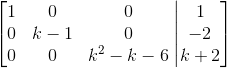# Suppose a system of equations is represented by a matrix, which has the following reduced row...

## Question:

Suppose a system of equations is represented by a matrix, which has the following reduced row echelon form:Find all values of k for which the solutions are:

(a) a plane

(b) a line

(c) a point

(d) the empty set

## System of Equations

A system of three equations with three unknowns can have a solution

with one free parameter (line), two free parameters ( plane), a unique solution (a point), or no solution (empty set).

If the augmented matrix of the system is given, then for a free parameter case, we need to have the case of a row with all entries zero.

For no solution, we need to have a case of a row with all entries zero, except the last entry.

(a) For a system of three equations with three unknowns, whose reduced row echelon form is given asto has the solution a plane, it means it needs to have two free-parameters or to have two rows that are satisfied by all values, like the form {eq}\displaystyle 0=0. {/eq}

Because the first and second row cannot be satisfied for any value, that means we don't have two rows of the form

{eq}\displaystyle 0=0, \implies \boxed{\text{ there is no }k \text{ such that the solution is a plane}}. {/eq}

(b) For the system to admit a line as a solution, it means it needs to have one free-parameter or one row that is satisfied by all values, like the form {eq}\displaystyle 0=0. {/eq}

Only the last row can provide a free parameter if

{eq}\displaystyle \begin{align} k^2-k-6=0 \text{ and }k+2=0\\ \iff k=-2, 3 \text{ and } k=-2 \\ \implies\boxed{ \text{ for }k=-2 \text{ the solution is a line }}. \end{align} {/eq}

(c) For the system to admit a point as a solution, it means the solution is unique, so the matrix can be reduced to the identity matrix, by dividing each row by its pivot,

so it needs

{eq}\displaystyle \begin{align} k^2-k-6\neq 0 \text{ and }k-1\neq 0\\ \iff k\neq -2, 3 \text{ and } k\neq 1 \\ \implies\boxed{ \text{ for any }k \text{ such that } k\neq -2, k\neq 1, k\neq 3 \text{ the solution is a point }}. \end{align} {/eq}

(d) For the system to admit no solution, or empty set, we need to have a row of zeros and the right hand side a nonzero.

For example, the second row

{eq}\displaystyle \begin{align} k-1 = 0 \iff k=1\\ \text{ or the last row }: k^2-k-6=0 \text{ and } k+2\neq 0 \iff k=3\\ \implies\boxed{ \text{ for }k=1 \text{ or } k=3 \text{ the solution is the empty set }}. \end{align} {/eq}